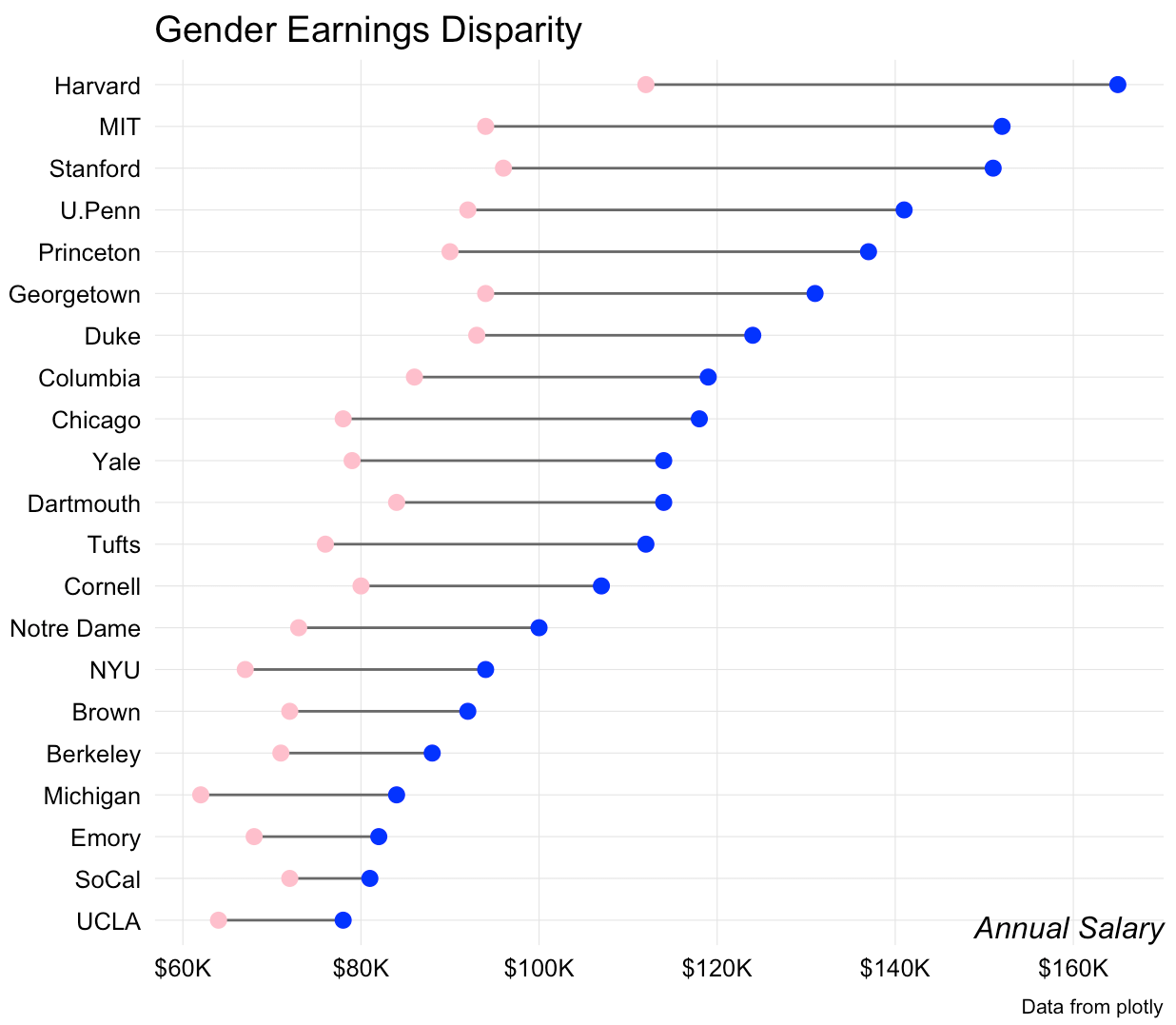Want to share your content on R-bloggers? click here if you have a blog, or here if you don't.

Shortly after I added lollipop charts to `ggalt` I had a few requests for a dumbbell geom. It wasn’t difficult to do modify the underlying lollipop `Geom`s to make a `geom_dumbbell()`. Here it is in action:

```library(ggplot2) library(ggalt) # devtools::install_github("hrbrmstr/ggalt") library(dplyr)   # from: https://plot.ly/r/dumbbell-plots/ URL <- "https://raw.githubusercontent.com/plotly/datasets/master/school_earnings.csv" fil <- basename(URL) if (!file.exists(fil)) download.file(URL, fil)   df <- read.csv(fil, stringsAsFactors=FALSE) df <- arrange(df, desc(Men)) df <- mutate(df, School=factor(School, levels=rev(School)))   gg <- ggplot(df, aes(x=Women, xend=Men, y=School)) gg <- gg + geom_dumbbell(colour="#686868", point.colour.l="#ffc0cb", point.colour.r="#0000ff", point.size.l=2.5, point.size.r=2.5) gg <- gg + scale_x_continuous(breaks=seq(60, 160, by=20), labels=sprintf("\$%sK", comma(seq(60, 160, by=20)))) gg <- gg + labs(x="Annual Salary", y=NULL, title="Gender Earnings Disparity", caption="Data from plotly") gg <- gg + theme_bw() gg <- gg + theme(axis.ticks=element_blank()) gg <- gg + theme(panel.grid.minor=element_blank()) gg <- gg + theme(panel.border=element_blank()) gg <- gg + theme(axis.title.x=element_text(hjust=1, face="italic", margin=margin(t=-24))) gg <- gg + theme(plot.caption=element_text(size=8, margin=margin(t=24))) gg```The API isn’t locked in, so definitely file an issue if you want different or additional functionality. One issue I personally still have is how to identify the left/right points (blue is male and pink is female in this one).

### Working Out With Dumbbells

I thought folks might like to see behind the ggcurtain. It really only took the addition of two functions to `ggalt`: `geom_dumbbell()` (which you call directly) and `GeomDumbbell()` which acts behind the scenes.

There are a few additional, custom parameters to `geom_dumbbell()` and the mapped `stat` and `position` are hardcoded in the `layer` call. We also pass in these new parameters into the `params` list.

```geom_dumbbell <- function(mapping = NULL, data = NULL, ..., point.colour.l = NULL, point.size.l = NULL, point.colour.r = NULL, point.size.r = NULL, na.rm = FALSE, show.legend = NA, inherit.aes = TRUE) {   layer( data = data, mapping = mapping, stat = "identity", geom = GeomDumbbell, position = "identity", show.legend = show.legend, inherit.aes = inherit.aes, params = list( na.rm = na.rm, point.colour.l = point.colour.l, point.size.l = point.size.l, point.colour.r = point.colour.r, point.size.r = point.size.r, ... ) ) }```

The exposed function eventually calls it’s paired `Geom`. There we get to tell it what are required `aes` parameters and which ones aren’t required, plus set some defaults.

We automagically add `yend` to the data in `setup_data()` (which gets called by the `ggplot2` API).

Then, in `draw_group()` we create additional `data.frame`s and return a list of three `Geom` layers (two points and one segment). Finally, we provide a default legend symbol.

```GeomDumbbell <- ggproto("GeomDumbbell", Geom, required_aes = c("x", "xend", "y"), non_missing_aes = c("size", "shape", "point.colour.l", "point.size.l", "point.colour.r", "point.size.r"), default_aes = aes( shape = 19, colour = "black", size = 0.5, fill = NA, alpha = NA, stroke = 0.5 ),   setup_data = function(data, params) { transform(data, yend = y) },   draw_group = function(data, panel_scales, coord, point.colour.l = NULL, point.size.l = NULL, point.colour.r = NULL, point.size.r = NULL) {   points.l <- data points.l\$colour <- point.colour.l %||% data\$colour points.l\$size <- point.size.l %||% (data\$size * 2.5)   points.r <- data points.r\$x <- points.r\$xend points.r\$colour <- point.colour.r %||% data\$colour points.r\$size <- point.size.r %||% (data\$size * 2.5)   gList( ggplot2::GeomSegment\$draw_panel(data, panel_scales, coord), ggplot2::GeomPoint\$draw_panel(points.l, panel_scales, coord), ggplot2::GeomPoint\$draw_panel(points.r, panel_scales, coord) )   },   draw_key = draw_key_point )```

In essence, this new geom saves calls to three additional `geom_`s, but does add more parameters, so it’s not really clear if it saves much typing.

If you end up making anything interesting with `geom_dumbbell()` I encourage you to drop a note in the comments with a link.